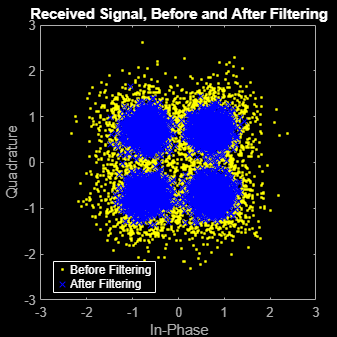# Interpolate and Decimate Using RRC Filter

This example shows how to interpolate and decimate signals using square-root, raised cosine filters designed with the `rcosdesign` function. This example requires the Communications Toolbox™ software.

Define the square-root raised cosine filter parameters. Define the signal constellation parameters.

```rolloff = 0.25; % Filter rolloff span = 6; % Filter span sps = 4; % Samples per symbol M = 4; % Size of the signal constellation k = log2(M); % Number of bits per symbol```

Generate the coefficients of the square-root raised cosine filter using the `rcosdesign` function.

`rrcFilter = rcosdesign(rolloff,span,sps);`

Generate 10000 data symbols using the `randi` function.

`data = randi([0 M-1],10000,1);`

Apply PSK modulation to the data symbols. Because the constellation size is 4, the modulation type is QPSK.

`modData = pskmod(data,M,pi/4);`

Using the `upfirdn` function, upsample and filter the input data.

`txSig = upfirdn(modData,rrcFilter,sps);`

Convert the Eb/N0 to SNR and then pass the signal through an AWGN channel.

```EbNo = 7; snr = EbNo + 10*log10(k) - 10*log10(sps); rxSig = awgn(txSig,snr,'measured');```

Filter and downsample the received signal. Remove a portion of the signal to account for the filter delay.

```rxFilt = upfirdn(rxSig,rrcFilter,1,sps); rxFilt = rxFilt(span+1:end-span);```

Create a scatterplot of the modulated data using the first 5000 symbols.

```hScatter = scatterplot(sqrt(sps)* ... rxSig(1:sps*5000), ... sps,0); hold on scatterplot(rxFilt(1:5000),1,0,'bx',hScatter) title('Received Signal, Before and After Filtering') legend('Before Filtering','After Filtering') axis([-3 3 -3 3]) % Set axis ranges hold off```